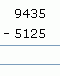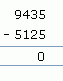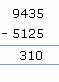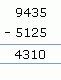Name: ___________________Date:___________________

 Email us to get an instant 20% discount on highly effective K-12 Math & English kwizNET Programs!

### Grade 3 - Mathematics6.4 Subtraction - 4 Digits

Method:

1. Start subtracting from the ones place.
2. If the subtrahend is lesser than minuend then we borrow 1 from tens place.
3. If the subtrahend is lesser than minuend in tens place then we borrow 1 from hundreds place.
4. If the subtrahend is lesser than minuend in hundreds place then we borrow 1 from thousands place.

Subtraction without regrouping:

Example 1:
 Question Step 1 Step 2 Step 3 Step 41. Start from ones place 5 minus 5 is 0.
2. In the tens place, 3 minus 2 is 1.
3. In the hundreds place 4 minus 1 is 3.
4. In thousands place, 9 minus 5 is 4.

Example 2:1. Start from ones place 8 minus 6 is 2.
2. In the tens place, 9 minus 9 is 0.
3. In the hundreds place 9 minus 8 is 1.
4. In thousands place, 4 minus 2 is 2.

Subtraction with regrouping:

Example:1. Start from ones place 7 minus 2 is 5.
2. In the tens place 3 is less than 8, so 1 is borrowed from 0 which has borrowed 1 from 3. So the result in tens place is 13 minus 8 which is 5.
3. In the hundreds place 0 becomes 9, the result is 3.
4. In thousands place, 3 becomes 2 and the result is 1.

Directions: Subtract the following.Name: ___________________Date:___________________

### Grade 3 - Mathematics6.4 Subtraction - 4 Digits

 Question 1: 8233 - 2371

 Question 2: 9554 - 3554

 Question 3: 8068 - 3835

 Question 4: 9347 - 4723

 Question 5: 7093 - 1989

Question 6: This question is available to subscribers only!

Question 7: This question is available to subscribers only!

Question 8: This question is available to subscribers only!

Question 9: This question is available to subscribers only!

Question 10: This question is available to subscribers only!